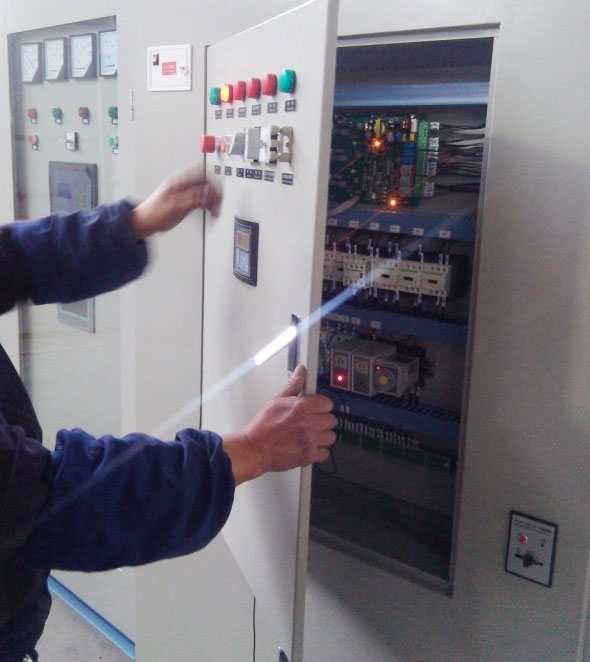Categories

# What is the soft starter standard setting for Motor Protection?

The current drawn at starting (starting current, also called "locked rotor" or "inrush") is directly proportional to the voltage applied at the terminals. This is why soft starters (also called "reduced voltage" starters) are used in the first place.

A reasonable assumption for "standard" squirrel cage induction machines is to assume that the motor will draw somewhere between 7X and 10X the rated line current if started direct-on-line at full rated voltage. As the machine speeds up, the current will drop toward rated line current, finally settling into the rating at operating speed. Thus a soft starter set for 0.80 PU volts will limit current draw to somewhere between 5.6X and 8.0X. Applying a lower voltage would reduce the current draw even further.

EXERCISE CAUTION! Developed torque is proportional to the square of the voltage ratio. So 0.80 PU volts only gives (0.8 * 0.8) = 0.64 PU torque. Check ALL points of the speed-torque curve to ensure proper acceleration.